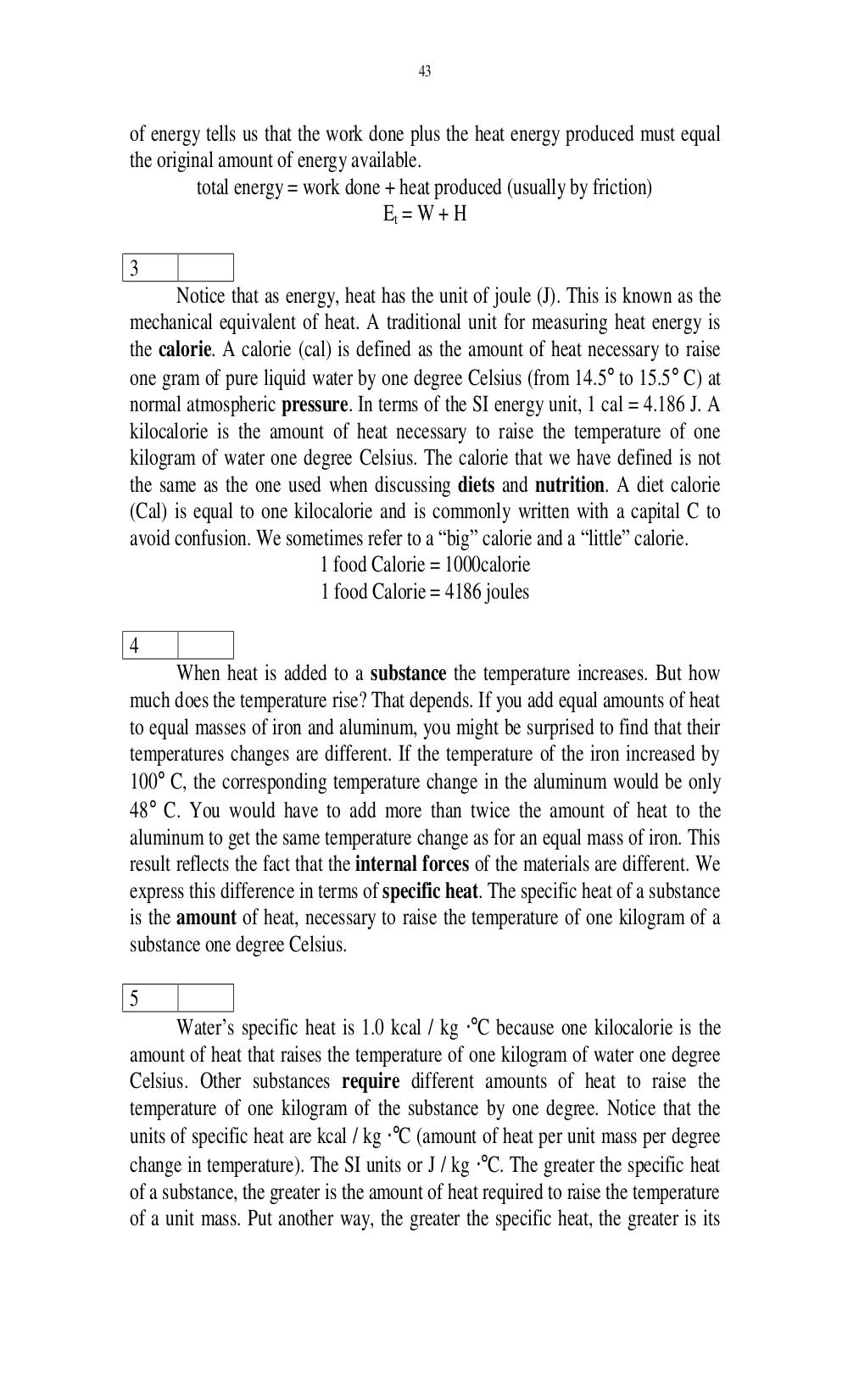# Английский язык. Ильичева Н.А - 43 стр.

Составители:

Рубрика:

• ## Иностранный язык43
of energy tells us that the work done plus the heat energy produced must equal
the original amount of energy available.
total energy = work done + heat produced (usually by friction)
E
t
= W + H
3
Notice that as energy, heat has the unit of joule (J). This is known as the
mechanical equivalent of heat. A traditional unit for measuring heat energy is
the calorie. A calorie (cal) is defined as the amount of heat necessary to raise
one gram of pure liquid water by one degree Celsius (from 14.5° to 15.5° C) at
normal atmospheric pressure. In terms of the SI energy unit, 1 cal = 4.186 J. A
kilocalorie is the amount of heat necessary to raise the temperature of one
kilogram of water one degree Celsius. The calorie that we have defined is not
the same as the one used when discussing diets and nutrition. A diet calorie
(Cal) is equal to one kilocalorie and is commonly written with a capital C to
avoid confusion. We sometimes refer to a big calorie and a little calorie.
1 food Calorie = 1000calorie
1 food Calorie = 4186 joules
4
When heat is added to a substance the temperature increases. But how
much does the temperature rise? That depends. If you add equal amounts of heat
to equal masses of iron and aluminum, you might be surprised to find that their
temperatures changes are different. If the temperature of the iron increased by
100° C, the corresponding temperature change in the aluminum would be only
48° C. You would have to add more than twice the amount of heat to the
aluminum to get the same temperature change as for an equal mass of iron. This
result reflects the fact that the internal forces of the materials are different. We
express this difference in terms of specific heat. The specific heat of a substance
is the amount of heat, necessary to raise the temperature of one kilogram of a
substance one degree Celsius.
5
Waters specific heat is 1.0 kcal / kg ⋅°C because one kilocalorie is the
amount of heat that raises the temperature of one kilogram of water one degree
Celsius. Other substances require different amounts of heat to raise the
temperature of one kilogram of the substance by one degree. Notice that the
units of specific heat are kcal / kg ⋅°C (amount of heat per unit mass per degree
change in temperature). The SI units or J / kg ⋅°C. The greater the specific heat
of a substance, the greater is the amount of heat required to raise the temperature
of a unit mass. Put another way, the greater the specific heat, the greater is its43

of energy tells us that the work done plus the heat energy produced must equal
the original amount of energy available.
total energy = work done + heat produced (usually by friction)
Et = W + H

3
Notice that as energy, heat has the unit of joule (J). This is known as the
mechanical equivalent of heat. A traditional unit for measuring heat energy is
the calorie. A calorie (cal) is defined as the amount of heat necessary to raise
one gram of pure liquid water by one degree Celsius (from 14.5° to 15.5° C) at
normal atmospheric pressure. In terms of the SI energy unit, 1 cal = 4.186 J. A
kilocalorie is the amount of heat necessary to raise the temperature of one
kilogram of water one degree Celsius. The calorie that we have defined is not
the same as the one used when discussing diets and nutrition. A diet calorie
(Cal) is equal to one kilocalorie and is commonly written with a capital C to
avoid confusion. We sometimes refer to a “big” calorie and a “little” calorie.
1 food Calorie = 1000calorie
1 food Calorie = 4186 joules

4
When heat is added to a substance the temperature increases. But how
much does the temperature rise? That depends. If you add equal amounts of heat
to equal masses of iron and aluminum, you might be surprised to find that their
temperatures changes are different. If the temperature of the iron increased by
100° C, the corresponding temperature change in the aluminum would be only
48° C. You would have to add more than twice the amount of heat to the
aluminum to get the same temperature change as for an equal mass of iron. This
result reflects the fact that the internal forces of the materials are different. We
express this difference in terms of specific heat. The specific heat of a substance
is the amount of heat, necessary to raise the temperature of one kilogram of a
substance one degree Celsius.

5
Water’s specific heat is 1.0 kcal / kg ⋅°C because one kilocalorie is the
amount of heat that raises the temperature of one kilogram of water one degree
Celsius. Other substances require different amounts of heat to raise the
temperature of one kilogram of the substance by one degree. Notice that the
units of specific heat are kcal / kg ⋅°C (amount of heat per unit mass per degree
change in temperature). The SI units or J / kg ⋅°C. The greater the specific heat
of a substance, the greater is the amount of heat required to raise the temperature
of a unit mass. Put another way, the greater the specific heat, the greater is its